SR #18: Light Cones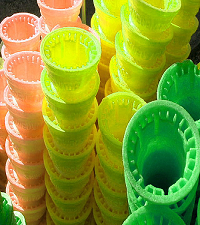Light cones?

Last time I focused on how it was possible for Al to see — even enclose in a tunnel — a train that appears shorter to him due to its motion. It turns out that the train Al sees is a stack of time slices of the train at different moments. As we've seen, lots of things look different in a moving frame.

Today I want to say a little about Em's point of view, run some numbers, and take you through a little math (just one equation, I promise). Then, because it's Friday (when I try to write about light), I'll introduce you to light cones.

They're not actually necessary, but they're kinda cool.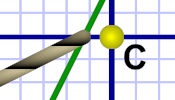Diagram 1. Shorter train!

The previous post considered an example where the train is the same length as the markers. When it passes Al at high speed, it appears foreshortened by an amount dependent on its speed relative to him.

Astute readers may have noticed something (especially if they kept on reading the latter part of the post): Even with a train only as long as the markers, and even given how the train appears foreshortened to Al, the snapshot of Em at the origin shows the train protruding beyond both markers.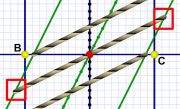Diagram 2. Longer train?

This is the same situation as when Em was halfway through the train tunnel, and this time the train is short enough to fit in the tunnel to begin with. So how is it that the ends seem to stick out?

Part of it has to do with the technical point I discussed at the end of the last post. It you skipped that, suffice to say the train shown in the diagram does appear to have grown rather than having contracted! The geometry of the diagrams requires it.

Remember that Al measures along horizontal lines, and Al's the one who sees a shorter train. Both diagram 1 and diagram 2 show the train's ends passing well inside the B & C markers where Al makes his measurement. So Al definitely sees a shorter train.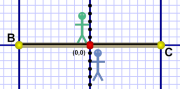Diagram 3. Marker measure.

But we know that the train and the markers have the same length. We used the (stopped) train to create the markers in the first place, so they have to be the same length. Al sees a shorter train because it's moving… what about Em — who's on the train?

According to Em, she and the train sit there while the world moves past (a perfectly valid view). That means — to her — the markers are moving, so the length between them is foreshortened. The markers appear closer together. Therefore, when Em is halfway between them, she sees the train protruding beyond them.

This is exactly what happened in the train tunnel (which was too short to begin with). Since the tunnel was foreshortened, Em saw the train sticking out even more.

Lets run some numbers! (Oh, come on, it'll be fun!)

Context Tunnel Train
Rest frame 6.0 meters 6.9 meters
Al's POV 6.0 meters 5.9 meters
Em's POV 5.2 meters 6.9 meters

So the train is normally longer than the tunnel, side-by-side. Al sees the train foreshortened to match the tunnel; Em sees the length difference get larger. These numbers are due to the speed: 0.5 c — which has a gamma factor (γ) of 1.1547.

We divide a normal length by gamma to give us the contracted length. Dividing the train's 6.9 meters by γ (1.1547) gives 5.98, so the train just fits inside the 6 meter tunnel. (And the tunnel: 6/γ = 5.2)

Let's consider the example from yesterday (also referenced above). If you count the grid squares, you find the train is 18 (let's call them) cubits long. As usual we're traveling at c/2, so our gamma is again 1.1547:

Context Markers Train
Rest frame 18.0 cubits 18.0 cubits
Al's POV 18.0 cubits 15.6 cubits
Em's POV 15.6 cubits 18.0 cubits

Because: 18/γ = 18/1.1547 = 15.6 cubits. Al sees the train shortened; Em sees the marker length shortened.

So now the question you may have is: What the heck is this gamma?

Gamma Factor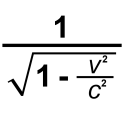gamma (γ)

The gamma factor, or just gamma, comes from the Lorentz equations that describe the transformations of Special Relativity. The gamma term appears in these equations where it quantifies the degree of change between frames.

It's the heart of Special Relativity!

The thing to notice about it is that the only thing that changes is v — the velocity (c is a constant and, obviously, so are the "1"s).

Given some speed, this equation gives you the gamma.

The interesting part is the v/c part (we can ignore squaring them for now). This ratio drives the term.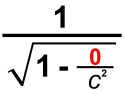v=0, therefore γ=1

If the velocity is zero, so is v/c. That means the lower part is square root of 1-0 or square root of one. Which is one. So the term becomes 1/1, or just one.

When you have no speed, your gamma is one.

If the velocity is the max possible (that is: c), then we have c/c, which gives us one. Now the lower part is square root of 1-1 or square root of zero. Which is zero.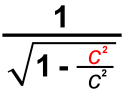v=c, therefore γ=?

And now there's a problem: the term became 1/0, which is  undefined. You can't divide by zero.

When you have max speed (c), your gamma is undefined! These equations can't be used to describe light's motion, only things moving slower than c.

As you speed up and approach c, your gamma, which started at one, grows — very slowly at first, but — eventually towards infinity as you approach light speed. As you get ridiculously close to light speed (talking 99.999999% of c) your gamma gets very high as it tends towards infinity.

This gamma term is part of the mathematical logic behind why nothing can approach light speed. The gamma term in the equations becomes undefined!

Light Cones

Every text on Special Relativity talks about light cones. While they're necessary for fully understanding SR, I find you don't actually need them to appreciate many of the interesting aspects.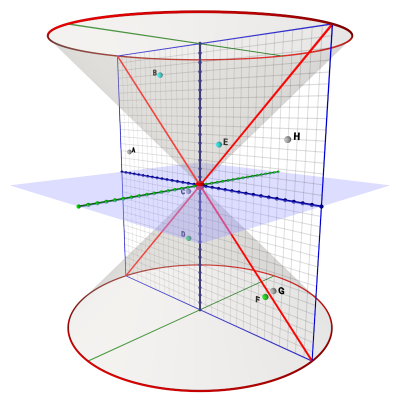Diagram 4. A light cone.

On the other hand, they do look cool! And they help bridge from our one-dimensional time-space diagrams to three-dimensional reality.

Light cones mostly live in a two-dimensional reality (not counting time). We imagine something like Flatland, but horizontal, flat, like a map. (The blue sheet in diagram 4, above.) And — just like a map — in this Flatland we can move east, west, north, or south.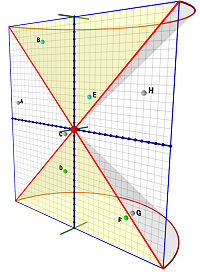Diagram 5. Cone slice.

The time dimension, as always, is the vertical dimension in our diagrams. With two spacial dimensions, plus one of time, these become 3D diagrams. Just remember that motion is horizontal and time is vertical.

The idea behind a light cone is that — for any given instant you want to call "now" — there extends a cone into your future representing light moving away from you.

It's a bit like the circular wave that moves away from you when you jump into the water. Think how that would look if the wave moved upward in time as it moved away from  you.

The angle of the cone is the angle of the motion of light: 45 degrees.

In fact, every diagram shown so far that had a light path line (usually red) showed a sliver of the cone. This whole time we've been dealing with a vertical slice through that cone.

There is also a lower cone that shows the path of light in your past reaching you at the "now" instant.Diagram 6. 2D light cone.

In our two-dimensional diagrams, there can be four light rays total. Two (one from each direction) extend upwards away from you. Two (one each direction) come from your past and converge on your "now" moment.

With a cone, beams can leave you or approach you from any point along the cone.

The key to a light cone is that anything inside the cone is reachable at sub-light speed. The edge of the cone represents light speed. Anything outside the cone is beyond reach — it requires faster-than-light travel to get there from the "now" point.

In diagram 6, signals from the origin point (red dot) can reach events B and E — they're within the future light cone. Signals can not reach events A or H, since it would require FTL.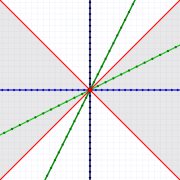My Special Relativity "icon" should make sense now!

Signals from events D and F can reach the origin — those events are in the past light cone. Event G is just barely outside, and event C (which is so close and so recent) is still outside the past light cone and doesn't make the cut.

The point is, things outside your light cones can't affect, or be affected by, you. Only things in your past light cone can affect you, and you can only affect things in your future light cone.

And on that note we wrap up the week!

Next week I'll discuss the relativity of time, and we'll fly around in spaceships. Some of us will grow older while others not so much (tip: try to get a ticket for the spaceship flights).

P.S.

Just as a visual treat (and possibly as instructive to those more into this), here are two 3D diagrams illustrating planes of simultaneity. With 2D drawings, the surface of simultaneity is a line; in a 3D drawing, it's a plane: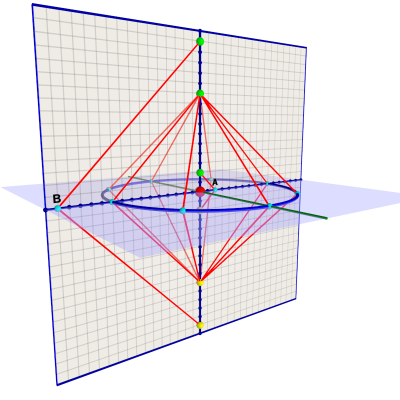Diagram 7. Al's plane of simultaneity.

We've seen how Al can bounce lasers off simultaneous events to his left and right. When the events are equally distant, the bounce is especially symmetrical.

In two dimensions, Al has a ring of points equally distant around him, and he can bounce his laser off any of them. Diagram 7 shows him bouncing eight laser beams off eight equally distant points equally spaced around him.

We see the same diamond symmetry we saw in the 2D diagrams.

Al also tested events A (much closer) and B (much further) to see if they were simultaneous. All Al's tests confirm his horizontal plane of simultaneity.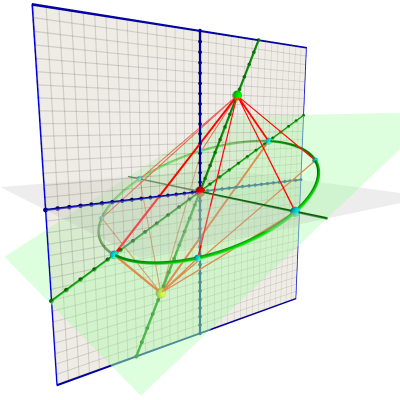Diagram 8. Em's plane of simultaneity.

Diagram 8 shows (Al's view of) Em bouncing eight lasers off points around her as she passes Al. (Em, of course, sees the same thing as Al sees in diagram 7.)

So, in 2D, Em's plane of simultaneity rotates (in Al's POV) due to her motion. (You can see her 1D line of simultaneity on the gray plane.) You can spend the weekend trying to imagine how a volume of simultaneity in our 3D world rotates due to motion!